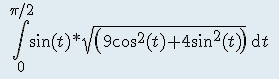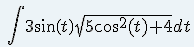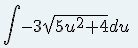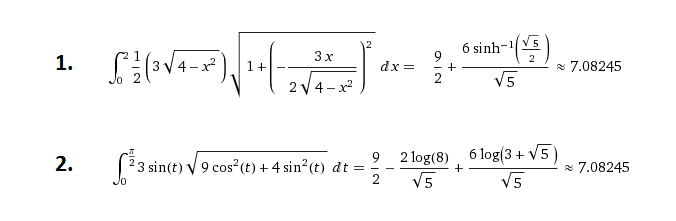# Hard integral

evol_w10lv

## Homework Statement

How to integrate:## The Attempt at a Solution

I used formula: sin^2(t) = ( 1-cos^2(t))
and now it's:Then:
u=cos(t)
du=-sin(t)What to do next?

Last edited:

## Answers and Replies

Homework Helper
Gold Member
Dearly Missed
We may now rewrite the integrand as:
$$-6\sqrt{s^{2}+1}, s=\frac{\sqrt{5}u}{2}$$
Now, utilize the trigonometric identity:
$$\tan^{2}(y)+1=\frac{1}{\cos^{2}(y)}$$
in a creative way.

•1 person
Gold Member
Where did the 3 come from?
Try using the identity 1 + tan2x = sec2x after some initial manipulation of the integrand.

•1 person
evol_w10lv
Actually the task sounds like this:Maybe I have to use polar coordinates? Any sugestions? Before I tried with diferent way, but I guess that integration without polar coordinates is too hard.

Homework Helper
Gold Member
Dearly Missed
L is a segment of an ellipse. You might go polar, but you can eliminate t as well, and express y as a function of x(Hint: sin^+cos^2=1)

evol_w10lvSeems to me that variant when we use y=y(x) is more complicated than variant with polar coordinates.

We may now rewrite the integrand as:
$$-6\sqrt{s^{2}+1}, s=\frac{\sqrt{5}u}{2}$$
Now, utilize the trigonometric identity:
$$\tan^{2}(y)+1=\frac{1}{\cos^{2}(y)}$$
in a creative way.

Not clear, how did you get there: $$s=\frac{\sqrt{5}u}{2}$$
We didn't learn about triple substitution, but I want to understand, how to get the final result. Can you explain some how?